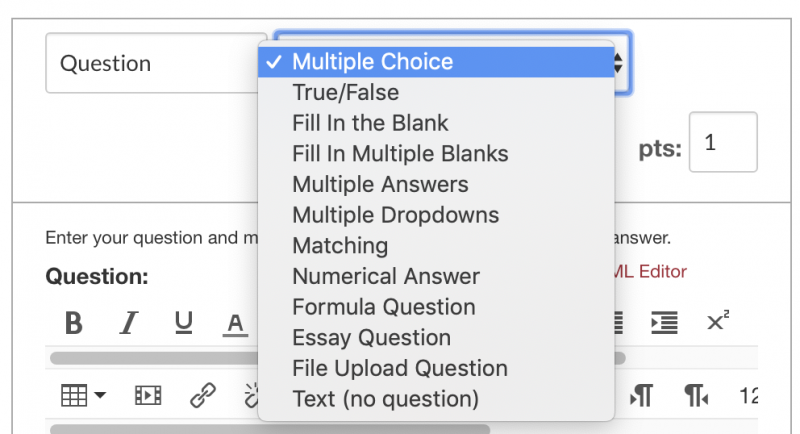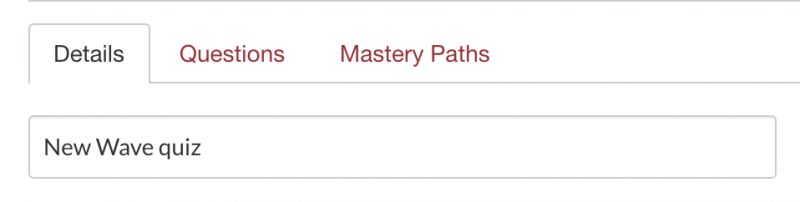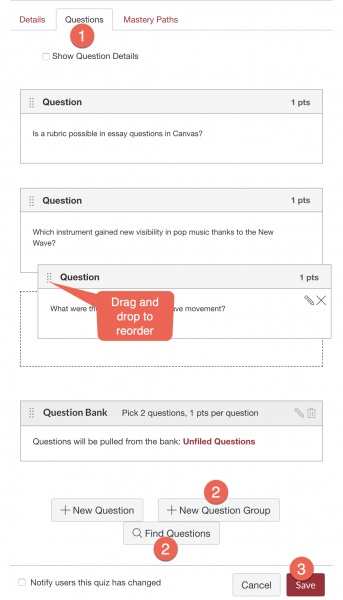# Canvas How-To: Quizzes

Note: the words "quiz," "test," "exam," etc. all represent the same thing in Canvas.  They are all configured using the Quiz tool.

## Creating a Quiz

In Canvas, quizzes are created in three phases:

1. Create questions in the Question Bank of the course. Do this for maximum flexibility in assembling the questions into a quiz. For any kind of randomization of questions during the quiz, question banks must be used.
2. Create the quiz shell with the details of the experience (dates, time limit, etc.)
3. Add questions to the shell by locating them in the Question Bank(s).

### Phase 1: Create Questions

From the Quizzes page in a course, open the action menu and choose Manage Question Banks. On that page, you can add a new question bank, and then create questions in the bank.

Question Banks are larger groups in which you may house multiple question items and types based on topics you need for your course.

Former Blackboard User Tip: Canvas's Question Bank performs the same function as Pools in Blackboard.

How do I create a Question Bank in a Course?

How do I use the Quizzes Index Page?

#### Canvas Question Types

Canvas has all of the most-common question types that were available in Blackboard.What kinds of question types can I create in Canvas?

### Phase 2: Configuring Quiz Details

Quizzes have two major settings to focus on: the Quiz Type and the Quiz Options. Canvas has four major Quiz Types for you to explore and then corresponding settings to enable their use. There are also many options that can be configured as desired, including time limits, multiple attempts, and open/close dates. Click Details to get started.Use the same Quiz tool for practice quizzes and various survey/questionnaire activities.What Quiz Types can I create in a Course?

What Options can I set in a Quiz?

### Phase 3: Adding Questions to a Quiz

Click the Questions tab on the Quiz settings page to begin adding questions to the quiz. Use this page to find questions in your question banks and add them to this quiz.  You can also add a Question Group, which will pull a random subset of questions from a selected question bank.

Drag and drop questions and question groups to reorder their appearance in the quiz.How do I create a Quiz by finding questions in a Question Bank?

How do I create a Quiz with a Question Group to randomize questions?

## Using Scientific Notation in Questions

Scientific notation is handled in various ways in quizzes, both in terms of instructors creating questions and students supplying answers. The section will break it down according to quiz question type.

### 1. Numeric questions

Numeric questions only accept numbers and number-related characters in the answer. The numbers are usually meant to be exact according to the correct answer, but instructors can configure answers in a few different ways.  See the full documentation for details.

#### Creating the question text

Instructors can type scientific notation directly into the question text as normal text using the regular text formatting tools, e.g., 6.02 x 1023.

There are three ways to specify correct answers in Numeric questions: Exact Answer, Answer in Range, and Answer with Precision. Use of scientific notation is different for the three choices. In all cases, both instructor and student must use 'e' notation (e.g., 6.02e23) to input scientific notation.

• Exact Answer & Answer in Range: Instructors can supply an answer in scientific notation if the exponent is 21 or greater. Numbers with exponents between 2 and 20 will be expanded to their decimal form after the instructor enters them. Students who type an exponent less than 21 will also have their answer expanded into decimal form.
• Negative exponents: Negative exponents in these two choices up to e-4 will be expanded into decimal form. Exponents e-5 and above will just be rendered as zero.
• Answer with Precision: Very complicated to use scientific notation in this setting, especially if the instructor wishes to preserve the notation as the basis for evaluating student input and as the style students will use to input their answer. Whether a number retains the scientific notation depends on the exponent and the amount of precision the instructor sets (i.e., significant figures).
• Negative exponents are buggy, so stick with postive exponents if possible.

### 2. Formula questions

Formula questions allow the instructor to set up variables that are automatically filled with unique values for each student. Students work with a formula to calculate their answers.

#### a. Creating the question text

Alongside the variable indicators, instructors can type scientific notation directly into the question text as normal text using the regular text formatting tools, e.g.,"What is [a] x 6.02 x 103?"  [a] represents a variable in that question.  Next, the instructor determines the min and max of the possible values for [a] as well as the formula Canvas will use to evaluate students responses.

#### b. Configuring the Variable Definition

Enter scientific notation using 1e2 format, which will be converted automatically to a decimal in the field, e.g., 100. Positive exponents are ok. For negative exponents, you can only use e-2.  e-3 or higher will be rendered as 0, even with the variable's Decimal Places set to 3.

#### c. Configuring the Formula Definition

Enter the scientific notation portion of the formula as 1*10^2. Here, Canvas retains the scientific format when you save the formula.  E.g., a*1*10^2.  Positive exponents are ok. For negative exponents, you can only go to 10^-6 as long as you also set the formula's Decimal Places menu to 4.  10^-7 and 'above' are just rendered as 0.

#### d. Student input

Students enter their answer using 1e2 format, but the number will be converted to decimal for exponents 20 and below. Exponents 21 and above will retain the scientific notation format.

Grading for quizzes of most preset question types is done atutomatically after students take their quiz. The Quiz Options allow you to set times or opportunities for students to view their answers and feedback. Essay type or file upload questions will require you to manually grade the content using SpeedGrader. You may wish to review our guides on using SpeedGrader if you have trouble.

Regrading quizzes because you might have accidently indicated the wrong correct answer in a question is primarily done by the question type. Use these guides to explore how to regrade:

What options can I use to Regrade a Quiz in a Course?

## Viewing Quiz Results

Quiz results are easily viewed and analyized using Canvas' Quiz Statistics feature. For detailed guides on how to use these tools and what some of the information means, please use the following guides:

How do I view student results in a Quiz?

How do I view a quiz log for a student?

## Giving extra quiz time

Students needing extra time because of special circumstance can be accommodated accordingly. You will use the Moderate this Quiz option after clicking on the quiz title. This is similar to the Availability Exception in Blackboard.  Different students can each be given different time extensions, if necessary.

How can I give my students extra time?